[an error occurred while processing this directive]

# Fields Demo/tutorial:

Some examples created by Doug Nychka using functions from the Fields software package designed to quickly familiarize users with some of the tools and methods available. For more organized coverage of this software, check out the Fields On-Line Manual.

Some color-coding makes the tutorial easier to follow, so here goes
(by convention, all comments are preceeded by a '#'):

These are commands   to faciliate "cutting&pasting", we omit the R prompt ('>')
These are output
Because we can, the graphics are included after the commands that generated them.

## Rats ...

This was experiment that involved feeding one group of rats an appetite supressant while the treatment was withheld from the contol group (naturally). The treatment was removed around day 65. The food intake in the control group is in `rat.diet\$con`. The food intake for the rat treatment group is `rat.diet\$trt`.

```
plot( rat.diet\$t,  rat.diet\$trt)

# complete experiment

matplot( rat.diet\$t, cbind( rat.diet\$trt, rat.diet\$con),
ylab="food intake", xlab="day")

title(" rat diet data: treatment and control groups")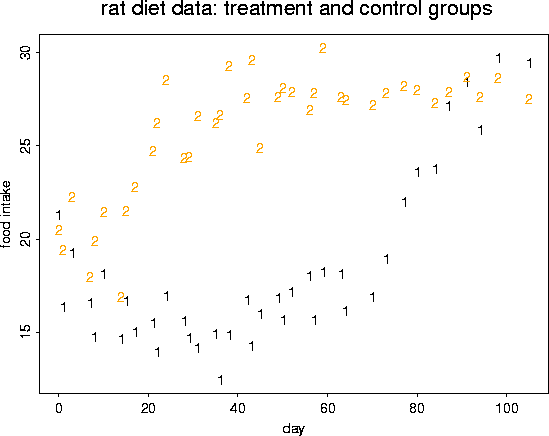# fit a nonparametric curve ( a cubic spline) to these data.
# curve has 4 degress of freedom

out <- sreg( rat.diet\$t, rat.diet\$trt, df=4)
out

Call:
sreg(x = rat.diet\$t, y = rat.diet\$trt, df = 4)

Number of Observations:       39
Effective degrees of freedom: 4
Residual degrees of freedom:  35
Residual root mean square:    1.433
Lambda ( smoothing parameter) 235.6

# take a look at the fit by adding a line to the current (active) figure

lines( rat.diet\$t, out\$fitted.values, col=1, lwd=2)# fit another spline (with 12 degrees of freedom)

out <- sreg( rat.diet\$t, rat.diet\$trt, df=12)
out

Call:
sreg(x = rat.diet\$t, y = rat.diet\$trt, df = 12)

Number of Observations:       39
Effective degrees of freedom: 12
Residual degrees of freedom:  27
Residual root mean square:    1.14
Lambda ( smoothing parameter) 1.202

lines( rat.diet\$t, out\$fitted.values, col=4, lwd=2)# now fit the spline using GCV to choose the model degrees of freedom

out <- sreg( rat.diet\$t, rat.diet\$trt)
out

Call:
sreg(x = rat.diet\$t, y = rat.diet\$trt)

Number of Observations:       39
Effective degrees of freedom: 7.5
Residual degrees of freedom:  31.5
Residual root mean square:    1.247
Lambda ( smoothing parameter) 11.07

plot( out, pch="*")# Plot GCV curve and its derivative by creating some temporary arrays.
# Note we can subdivide the plot window

x     <- seq( 0, 100,, 80)
hold  <- predict( out, x)
hold2 <- predict( out, x, derivative=1)

set.panel(2,1)

plot( rat.diet\$t, rat.diet\$trt)
lines( x, hold)

plot( x, hold2, xlab="days", ylab="change in intake rate", type="l")# To get some standard errors, it is easier to switch to the
# thin plate spline function ...  Tps

out <- Tps( rat.diet\$t, rat.diet\$trt)
out

Call:
Tps(x = rat.diet\$t, Y = rat.diet\$trt, cov.function = "Thin plate spline radial

Number of Observations:                        39
Degree of polynomial null space ( base model): 1
Number of parameters in the null space         2
Model degrees of freedom:                      7.5
Residual degrees of freedom:                   31.5
GCV estimate for sigma:                        1.387
MLE for sigma:                                 1.356
lambda                                         0.00037
rho                                            4936
sigma^2                                        1.839

set.panel( 1,1)
fit <- predict( out, x)
se  <- predict.se( out, x)

# plot data,
# GCV spline, and
# upper and lower pointwise CI's

plot( rat.diet\$t, rat.diet\$trt, ylim=c(12,32), pch="*")
lines( x, fit, lwd=2)
lower <- fit - 1.96* se
upper <- fit + 1.96*se
lines(x, lower, lty=2)
lines(x, upper, lty=2)

# Bonferoni bounds

bonf   <- qnorm( .025/39)
blower <- fit - bonf* se
bupper <- fit + bonf*se
lines(x, blower, lty=3, col=2)
lines(x, bupper, lty=3, col=2)

# and now for the control group of rats.
# (same strategy as we used for the trial group of rats)

out <- Tps( rat.diet\$t, rat.diet\$con)
out

Call:
Tps(x = rat.diet\$t, Y = rat.diet\$con, cov.function = "Thin plate spline radial

Number of Observations:                        39
Degree of polynomial null space ( base model): 1
Number of parameters in the null space         2
Model degrees of freedom:                      7.6
Residual degrees of freedom:                   31.4
GCV estimate for sigma:                        1.579
MLE for sigma:                                 1.521
lambda                                         0.00034
rho                                            6769
sigma^2                                        2.314

fit <- predict( out, x)
se  <- predict.se( out, x)

points( rat.diet\$t, rat.diet\$con, pch="o")
lines( x, fit, lwd=2)

lower <- fit - 1.96* se
upper <- fit + 1.96*se
lines(x, lower, lty=2)
lines(x, upper, lty=2)

bonf   <- qnorm( .025/39)
blower <- fit - bonf* se
bupper <- fit + bonf*se
lines(x, blower, lty=3, col=2)
lines(x, bupper, lty=3, col=2)# what is a spline doing to the data?

out  <- Tps( rat.diet\$t, rat.diet\$trt )
A    <- make.Amatrix( out )
look <- A%*% rat.diet\$trt

# predicted from the hat matrix times y

look2 <- predict( out )

# predicted from the Tps object
# list them out to compare

cbind( look,look2)

# the rows of A are the weights applied to the observed data to
# give the spline at the points

row20 <- c(A[20,])
row35 <- c( A[35,])
matplot( rat.diet\$t , cbind( row20, row35), type="l", col=1)
yline( 0)```

## Ozone ...

```# lets load the ozone2 dataset and make a map
# map of ozone data sites

data(ozone2)
names(ozone2)

 "lon.lat"        "y"              "chicago.subset"

US( xlim= c(-93.6,-82.8),  ylim=c(36.7, 44.5) )
points( ozone2\$lon.lat, pch="o")# take a look at day 16:  June 18, 1987
# removing the missing values complicates things a bit, but not much.

y16.full <- c(ozone2\$y[16,])
good     <- !is.na(y16.full)
y16      <- y16.full[good]
x        <- ozone2\$lon.lat[good,]

# OK now we have removed the missing values for day 16 and we
# called it "y16", their locations are in "x"
# thin plate spline

out <- Tps( x, y16 )
out

Call:
Tps(x = x, Y = y16, cov.function = "Thin plate spline radial basis functions

Number of Observations:                        147
Degree of polynomial null space ( base model): 1
Number of parameters in the null space         3
Model degrees of freedom:                      74.6
Residual degrees of freedom:                   72.4
GCV estimate for sigma:                        9.275
MLE for sigma:                                 8.392
lambda                                         0.00014
rho                                            489200
sigma^2                                        70.43

surface( out)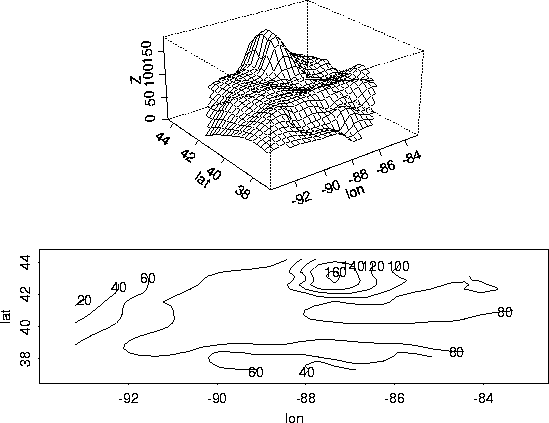# try out some other spatial covariances
# One example of a covariance function is the exponential:
# exp.cov or exp.cov.S

out <- Krig( x, y16, exp.cov, theta=2 )
surface(out)# some more complicated plots

look <- predict.surface( out )
image.plot(look, graphics.reset=F )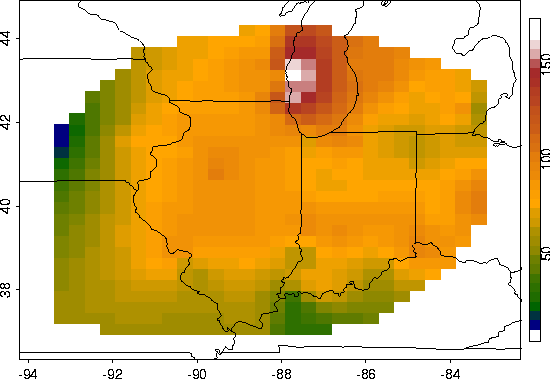# a better covariance

set.panel()
out <- Krig( x, y16, exp.earth.cov, theta=343)
plot(out)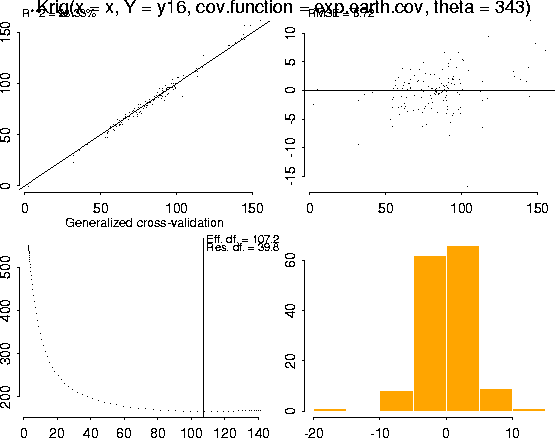surface(out)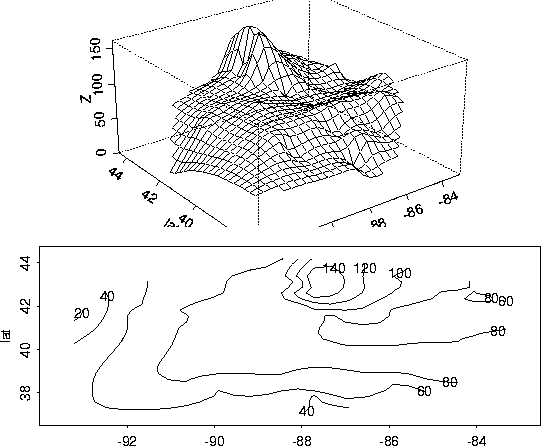# find some time averaged fields

ozone.cor <- COR( ozone2\$y)
mean.tps  <- Tps( ozone2\$lon.lat, ozone.cor\$mean,
return.matrices=F)
sd.tps    <- Tps( ozone2\$lon.lat, ozone.cor\$sd,
return.matrices=F)

# make up a data set of pairwise distance by correlation.

upper    <- col(ozone.cor\$cor) > row(ozone.cor\$cor)
cgram.oz <- list( d=rdist.earth( ozone2\$lon.lat)[upper],
cor=ozone.cor\$cor[upper])

# plot correlations as a function of distance

plot( cgram.oz\$d, cgram.oz\$cor, xlab="correlation",
ylab="distance",pch=".")
title( "sample correlation as function of distance")# this is where the 343 came from for the range parameter

library(nls)
cgram.fit <- nls( cor ~ alpha* exp( -d/theta),
cgram.oz, start= list( alpha=.95, theta=200))
summary( cgram.fit)

Formula: cor ~ alpha * exp(-d/theta)

Parameters:
Estimate  Std.Error t value   Pr(>|t|)
alpha 9.306e-01  3.701e-03   251.5   <2e-16 ***
theta 3.434e+02  2.403e+00   142.9   <2e-16 ***
---
Signif. codes:  0 `***' 0.001 `**' 0.01 `*' 0.05 `.' 0.1 ` ' 1

Residual standard error: 0.1216 on 11626 degrees of freedom

Correlation of Parameter Estimates:
alpha
theta -0.8384

# OK now we are going to fit the "right" model.
# We standarize the ozone and fit a surface to the standardized data
# (and undo things for predicting and inference)

fit.GCV <- Krig( x, y16, cov.function=exp.earth.cov,theta=343,
sd.obj=sd.tps, mean.obj= mean.tps, m=2)

summary( fit.GCV)

CALL:
Krig(x = x, Y = y16, cov.function = exp.earth.cov, m = 2, mean.obj = mean.tps,
sd.obj = sd.tps, theta = 343)

Number of Observations:                        147
Number of unique points:                       147
Degree of polynomial null space ( base model): 1
Number of parameters in the null space         3
Effective degrees of freedom:                  115.6
Residual degrees of freedom:                   31.4
MLE sigma                                      0.2859
GCV est. sigma                                 0.3129
MLE rho                                        7.698
Scale used for covariance (rho)                7.698
Scale used for nugget (sigma^2)                0.08175
lambda (sigma2/rho)                            0.01062

RESIDUAL SUMMARY:
min     1st Q    median     3rd Q       max
-0.593400 -0.075240  0.002854  0.079600  0.422300

COVARIANCE MODEL: exp.earth.cov
A correlation model was fit: Y is standardized
before
spatial estimate is found

DETAILS ON SMOOTHING PARAMETER:
Method used:      Cost:  1
lambda       trA       GCV   GCV.one GCV.model      shat
0.01062 115.63608   0.45895   0.45895        NA   0.31292

Summary of estimates for lambda
lambda   trA    GCV   shat
GCV     0.01062 115.6 0.4590 0.3129
GCV.one 0.01062 115.6 0.4590 0.3129

# the finale

look  <- predict.surface( fit.GCV )
look2 <- predict.surface.se( fit.GCV )
set.panel()
par( pty="s")

image.plot( look, graphics.reset=F)